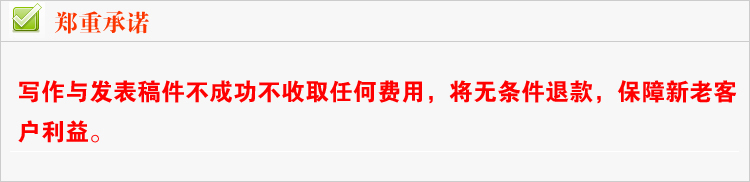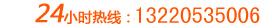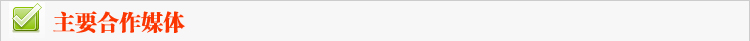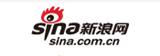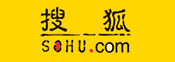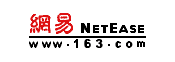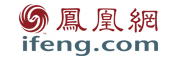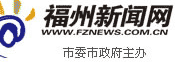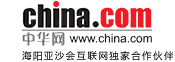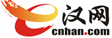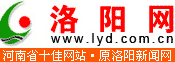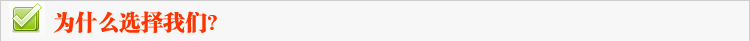⊙使受众“眼软”

⊙使受众“心软”

⊙使受众“脑软”1.1

2.2

3.3

⊙重大企业事件
⊙参与慈善活动
⊙行业特色事件
⊙危机公关事件

1.1

2.2

3.3

⊙人物新闻访谈
⊙发表行业性观点
⊙社会热点点评
⊙荣誉及社会责任

1.1

2.2

3.3# 我要留言

 客户姓名： 留言主题： 客户评价：好评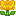中评差评 留言内容： 贵站代写的软文质量真是帮了我的大忙了，经过这段时间的验证，你们帮我发表的软文很有成效，业务明显增加，谢谢你们的原创写作！希望可以继续与贵站合作！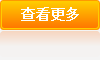<% sql_="select * from J_liuyan where shenhe=1 order by addtime desc, id desc" set rs_=conn.execute(sql_) for i=1 to 10 if rs_.eof then exit for %> <% rs_.movenext next %>
 客户： <%=rs_("username")%> <% if rs_("classid")=1 then%>好评<% end if %> <% if rs_("classid")=2 then%>中评<% end if %> <% if rs_("classid")=3 then%>差评<% end if %> ','','width=500,height=400')"><%=rs_("title")%>